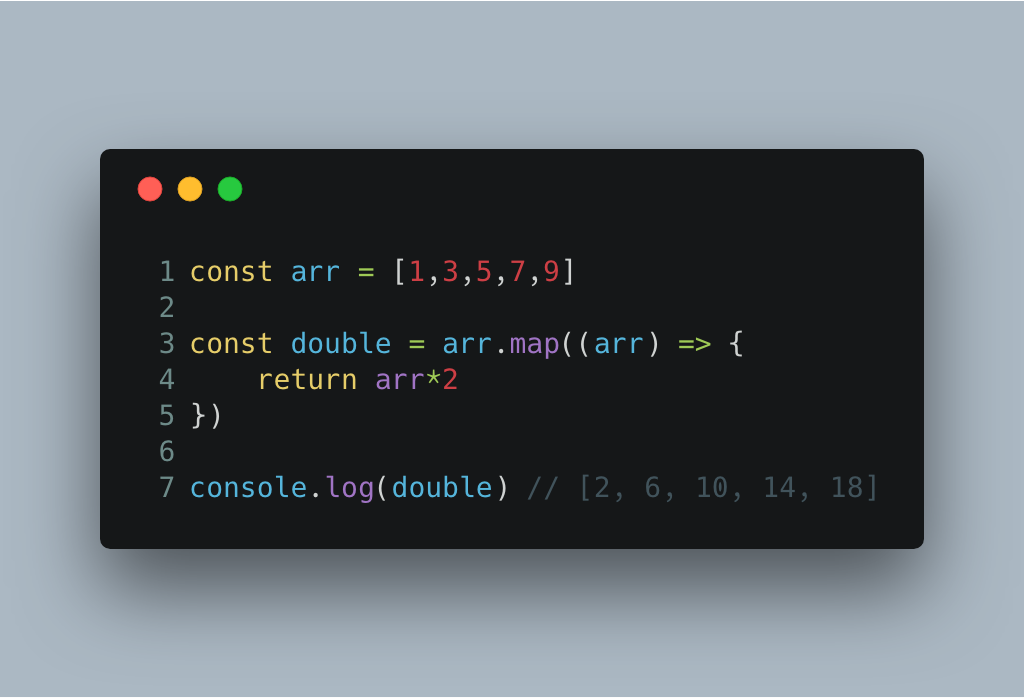# ES6 學習筆記（一） 箭頭函數### 範例

``````const arr = [1,3,5,7,9]

const double = arr.map(function(arr){
return arr*2
})

console.log(double) // [2, 6, 10, 14, 18]

//箭頭函數
const arr = [1,3,5,7,9]

const double = arr.map((arr) => {
return arr*2
})

console.log(double) // [2, 6, 10, 14, 18]
``````

``````const double = arr.map( arr => {
return arr*2
})
``````

``````const arr = [1,3,5,7,9]

const double = arr.map(() => {
return 'hello'
})

console.log(double) // ["hello", "hello", "hello", "hello", "hello"]
``````

``````const arr = [1,3,5,7,9]

const double = arr.map((arr) => arr*2)

console.log(double) // [2, 6, 10, 14, 18]
``````

``````const sayMyName = name => console.log(`you're \${name}`)

sayMyName('heisenberg') // you're heisenberg
``````